# Generating Reflectance Curves from sRGB Triplets (Page 8)

#### Comparison of Methods

(Update 1/9/20: The newly-developed LHTSS method presented below is a better choice than ILLSS when requiring the reflectance curves to fall strictly between 0 and 1. It matches the Munsell colors better and takes about 60% of the computational effort of ILLSS. See the 6/4/2019 update below for more information.)

I ran each of the five algorithms with every sRGB value (by fives) and have summarized the results in the table below. The total number of runs for each solution was 140,608.

 Name Execution Time (sec) Max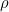MinNum>1 Num<0 Max Iter. Mean Iter. Computational Effort LLS, Linear Least Squares 2.7 1.36 -0.28 26,317 50,337 n/a n/a Matrix mult only LSS, Least Slope Squared 2.7 1.17 -0.17 9,316 48,164 n/a n/a Matrix mult only ILSS, Iterative Least Slope Squared 25.7 1 0 0 0 5 1.49 Mult soln of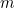linear eqns** LLSS, Least Log Slope Squared 322. 3.09 0 38,445 0 16 6.77 Mult soln of 39 linear eqns ILLSS, Iterative Least Log Slope Squared 525. 1 0 0 0 5* 1.41* Mult soln of (39+) linear eqns**

* This is outer loop iteration count. The inner loop has iteration count similar to previous line.
** The quantityis the number of reflectance values that end up being constrained at either 1 or 0.

Explanation of Columns

• Execution Time: Real-time duration to compute 140,608 reflectance curves of all sRGB values (in intervals of five), on a relatively slow Thinkpad X61 tablet. Relative times are more important than absolute times.
• Max: The maximum reflectance value of all computed curves.
• Min: The minimum reflectance value of all computed curves. Zero is used to represent some small specified lower bound, typically 0.0001.
• Num>1: The number of reflectance curves with maxima above 1.
• Num<0: The number of reflectance curves with minima below 0.
• Max Iter.: The largest number of iterations required for any reflectance curve (for iterative methods).
• Mean Iter.: The mean value of all iteration counts (for iterative methods).
• Computational Effort: Comments on the type of computation required for the method.

Clearly, the methods that don’t need to solve linear systems of equations are much faster. The reflectance curves they create, however, may not be very realistic.

#### Comparison of Reflectance Curves

In this section, I compare the computed reflectance curves against two large sets of measured reflectance data. My goal is to assess how “realistic” the computed reflectance curves are in comparison to reflectances measured from real colored objects.

Munsell Color Samples

The first set comes from the Munsell Book of Colors, specifically the 2007 glossy version. The Munsell system organizes colors by hue, chroma (like saturation), and value (like lightness). In 2012, Paul Centore measured 1485 different Munsell samples and published the results here. I computed the sRGB values of each sample, and used those quantities to compute reflectance curves for each of the five methods described above. Of the 1485 samples, 189 of them are outside the sRGB gamut (have values <0 or >255) and were not examined in this study, leaving 1296 in-gamut samples. An Excel file of the 1485 reflectance curves and sRGB values is available here.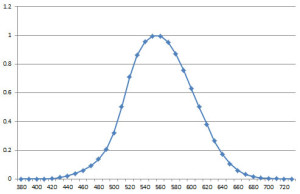The luminous efficiency curve, which describes the relative sensitivity of the eye to spectral light across the visible spectrum.

When comparing reflectance curves, some parts of the curve are more important than others. As we approach both ends of the visible spectrum, adjacent to the UV and IR ranges, the human eye’s sensitivity to these wavelengths rapidly decreases. This phenomenon is described by the “luminous efficiency” curve shown in the adjacent figure. As I was computing the reflectance curves, I noticed that there are often large discrepancies between the computed and measured reflectance curves at the ends of the visible spectrum. These large differences have relatively little impact on color perception, and would also have little consequence on operations performed on the computed reflectance curves, such as when modeling subtractive color mixture. Consequently, I decided to downplay these end differences when comparing the curves.

To assess how well each computed reflectance matches the measured reflectance curve, I first subtracted one from the other and took absolute values of the difference. Then I multiplied the differences by the luminous efficiency curve. Finally, I summed all of the values to give a single measure of how well the reflectance curves match, or a “reflectance match measure” (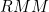):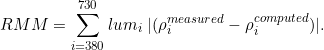Lower values ofrepresent a better match, with zero being a perfect match of the two reflectance curves. Keep in mind that regardless of the value of, the sRGB triplets of the computed and measured curves always match exactly, as would the perceived color evoked by the two reflectance curves.

I examined the maximum and mean values offor each of the five methods when applied to all 1296 Munsell samples:

 Name MaxMeanLLS, Linear Least Squares 2.46 0.88 LSS, Least Slope Squared 1.11 0.17 ILSS, Iterative Least Slope Squared 1.04 0.16 LLSS, Least Log Slope Squared 0.92 0.15 ILLSS, Iterative Least Log Slope Squared 0.86 0.15

The Linear Least Squares method is clearly much worse than the others. Considering that the Least Slope Squared method (LSS) requires the same computational effort as LLS, but with much better results, I decided to present the comparison figures that follow for the last four methods only: LSS, ILSS, LLSS, and ILLSS.

The following figures compare the four methods. Each gray square represents one Munsell sample, and the shade of gray indicates the correspondingvalue. The shade of gray is linearly mapped so that it is white for= 0 and black for= 1.11, the maximum valuefor all four methods.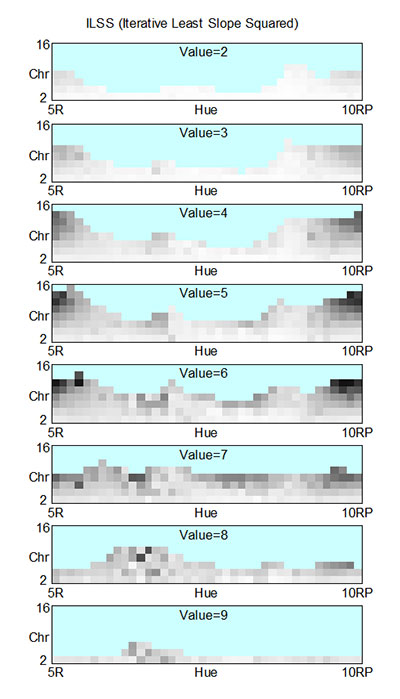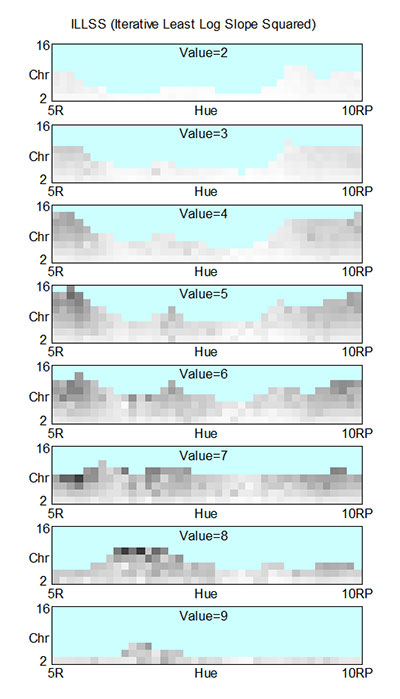Overall, the log version of the algorithms do a little better. The worst matches with non-log-based LSS/ILSS take place with the highly chromatic reds and purples, and sometimes with the bright yellows. With log-based LLSS/ILLSS, the worst matches tend to be in the bright yellows, and sometimes in the chromatic reds and oranges. In both pairs, the iterative version clips the reflectance curves between 0 and 1, and usually reduces the mismatch somewhat, but not by very much.

Here are some examples of LSS and ILSS with highs:This is typical of the mismatch with chromatic reds and purples. This one (Hue=5RP, Value=6, Chroma=12) has anof 1.04. The curve for LSS is not visible because it is directly behind the ILSS curve. There was no clipping needed, so the two curves are the same.

One weakness of the LSS method is that it tends to drop into the negative region for chromatic red colors. In these cases, the clipping provided by the ILSS method greatly improves the match. The following example has an LSSof 1.01 and an improved ILSSof 0.40: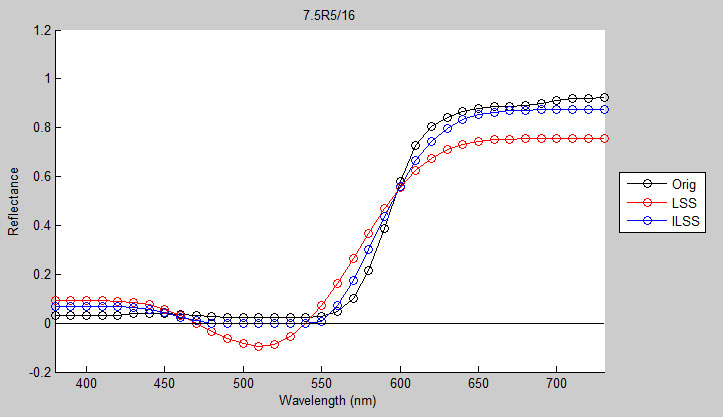The following is a typical mismatch for LSS/ILSS in the yellow region: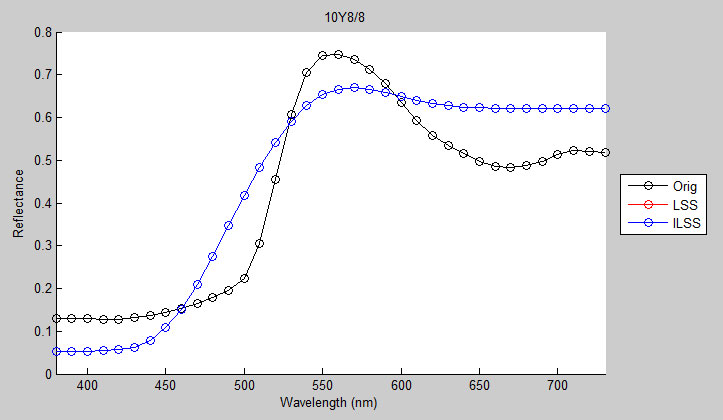Generally, when LSS/ILSS has a bad match, it is because it oscillates too little.

Here are some examples of log-based LLSS/ILLSS with highs: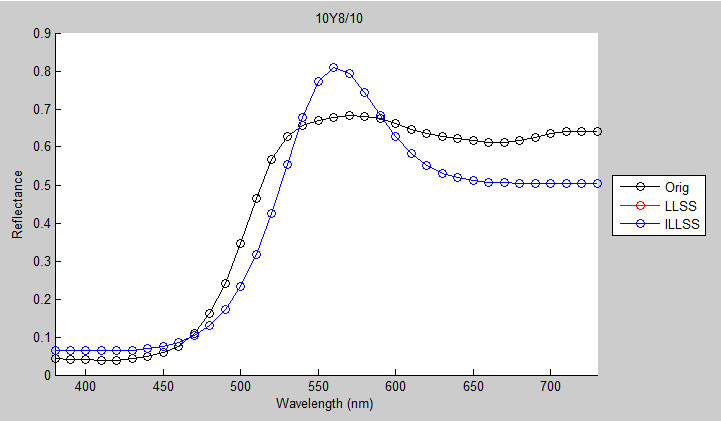This example has anof 0.88, mainly because it takes advantage of the wavelengths of light that give rise to a yellow sensation, in contrast to how the pigments in this sample get the same yellow sensation from a broader mix of wavelengths.

On bright red and orange Munsell samples, the LLSS/ILLSS curves tend to shoot high on the red side: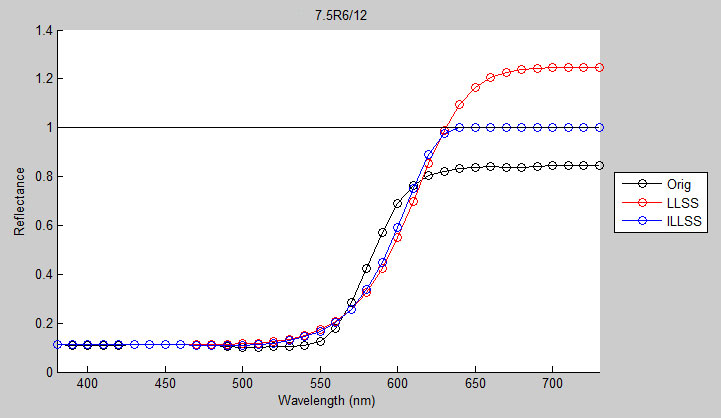Even though there is considerable clipping with the ILLSS version, most of it takes place in the long wavelengths, which does not help thescore as much. This is evident in how little ILLSS needs to adjust the mid-wavelength range to make up for the huge difference in the long wavelengths, while maintaining the same sRGB values.

In summary, the non-log versions (LSS/ILSS) tend to undershoot peaks and the log versions (LLSS/ILLSS) tend to overshoot them. Overall, the log versions give a somewhat better match, but at the expense of considerably more computation.

Commercial Paints and Pigments

The second large dataset of reflectance curves comes from Zsolt Kovacs-Vajna’s RS2color webpage. He has a database of reflectance curves for many sets of commercial paints and pigments, which can be obtained by emailing a request to him. They are grouped into the following families:

1. apaFerrario_PenColor
2. Chroma_AtelierInteractive
3. ETAC_EFX500b
4. ETAC_EFX500t
5. GamblinConservationColors
6. Golden_HB
7. Golden_OpenAcrylics
8. GretagMacbethMini
9. Holbein_Aeroflash
10. Holbein_DuoP
11. KremerHistorical
12. Liquitex_HB
13. Maimeri_Acqua
14. Maimeri_Brera
15. Maimeri_Polycolor
16. MunsellGlossy5B
17. MunsellGlossy5G
18. MunsellGlossy5P
19. MunsellGlossy5R
20. MunsellGlossy5Y
21. MunsellGlossyN
22. pigments
23. supports
24. Talens_Ecoline
25. Talens_Rembrandt
26. Talens_VanGoghH2Oil
27. whites
28. WinsorNewton_ArtAcryl
29. WinsorNewton_Artisan
30. WinsorNewton_Finity
31. WinsorNewtonHandbook

In total, there are 1493 different samples in these 31 groups. I discarded the 275 of them that were out of the sRGB gamut, and computed reflectance curves from the sRGB values of the remaining 1218 samples. I again computedvalues to compare the computed curves to the actual measured curves. The following animated GIFs show thevalues (color coded according to the colormap on the right) plotted in sRGB space.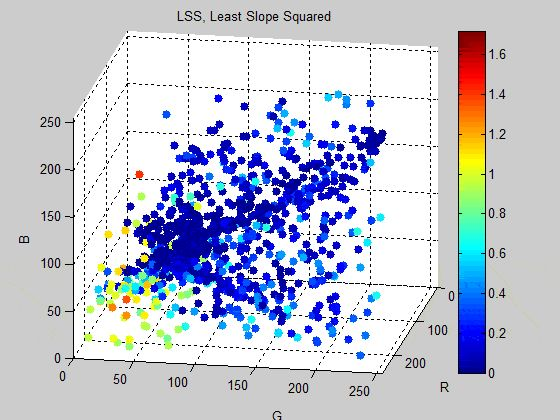Above,values (by color) for the LSS method plotted in sRGB space.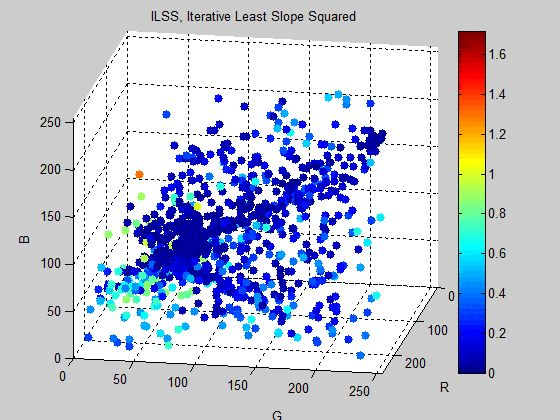Above,values (by color) for the ILSS method plotted in sRGB space.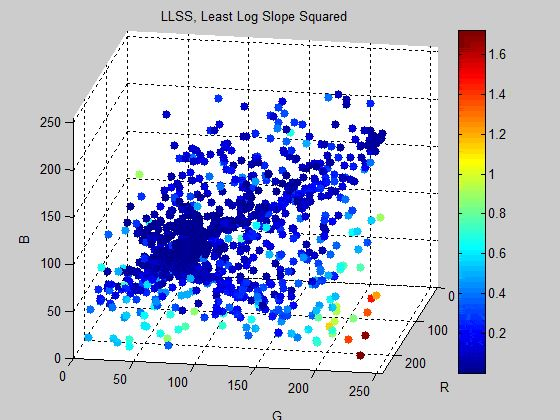Above,values (by color) for the LLSS method plotted in sRGB space.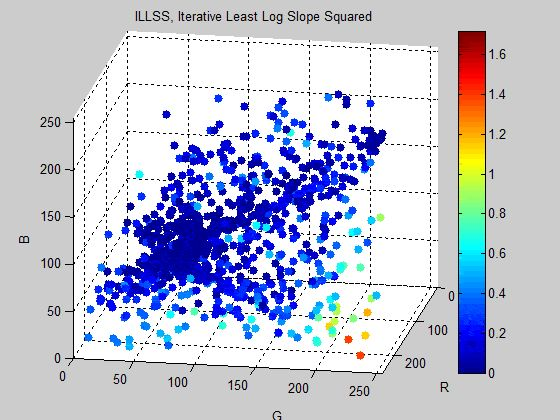Above,values (by color) for the ILLSS method plotted in sRGB space.

It is apparent from these GIFs that the non-log-based methods have a fairly large region of mismatch in the red region (large R, small G and B). The log-based versions have a much smaller region of mismatch in the yellow region (large R and G, small B). The iterative version of both methods improve the matches in both cases. The relative sizes of the mismatch regions can be seen more clearly in a projection onto the R-G plane:Above,values (by color) for the LSS method projected onto the sRGB R-G plane.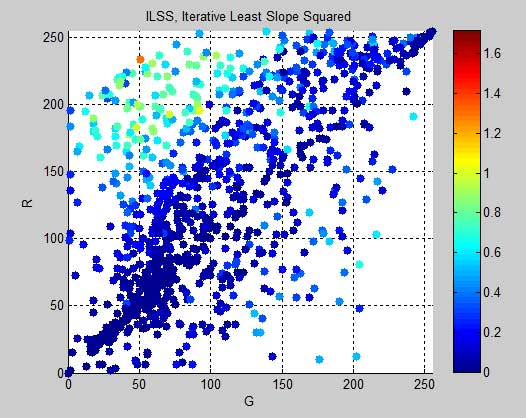Above,values (by color) for the ILSS method projected onto the sRGB R-G plane.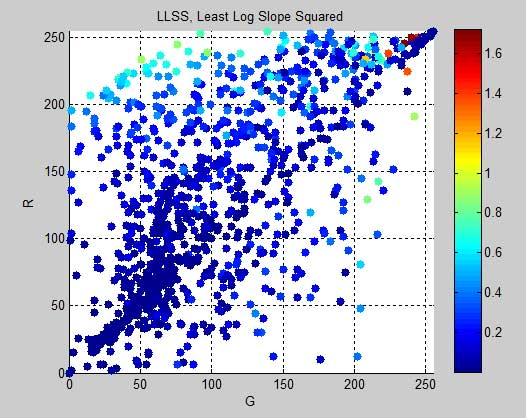Above,values (by color) for the LLSS method projected onto the sRGB R-G plane.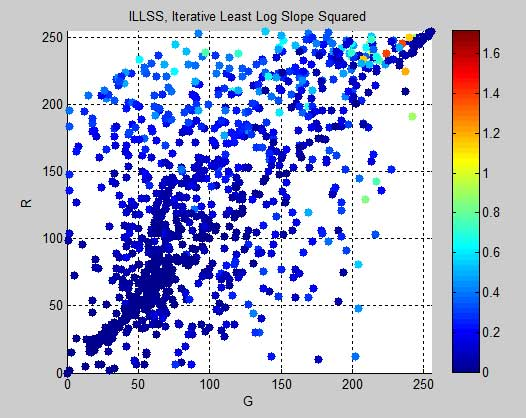Above,values (by color) for the ILLSS method projected onto the sRGB R-G plane.